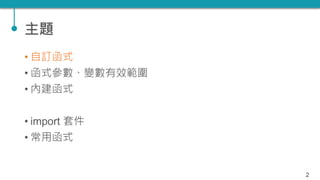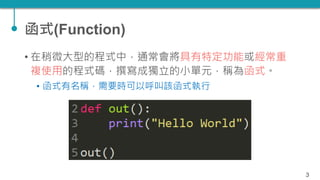Successfully reported this slideshow.

# TQC+ 程式語言 Python 05：函式

1

Share×
1 of 43
1 of 43

# TQC+ 程式語言 Python 05：函式

1

Share

#### Transcript

1. 1. 05：函式 2018.1.30 財團法人中華民國電腦技能基金會 策劃 逢甲大學 林英志 老師 編著
2. 2. 主題 • 自訂函式 • 函式參數、變數有效範圍 • 內建函式 • import 套件 • 常用函式 2
3. 3. 函式(Function) • 在稍微大型的程式中，通常會將具有特定功能或經常重 複使用的程式碼，撰寫成獨立的小單元，稱為函式。 • 函式有名稱，需要時可以呼叫該函式執行 3
4. 4. 函式 • 使用函式的程式設計方式有下列好處： • 將大程式切割後由多人撰寫，有利於團隊分工，可縮短程式開 發的時間。 • 可縮短程式的長度，程式碼也可重複使用，當再開發類似功能 的產品時，只需稍微修改即可以套用。 • 程式可讀性高，易於除錯和維護 4
5. 5. 自訂函式 • 建立函式的語法為： • def 函式名稱([ 參數1, 參數2, … ]): • 程式區塊 • [ return 回傳值1, 回傳值2, … ] 5
6. 6. 自訂函式 • 函式建立後並不會執行，必須在程式中呼叫函式才會執 行，呼叫函式的語法為： • [ 變數= ] 函式名稱([ 參數串列 ]) 6
7. 7. 參數預設值 • 建立函式時可以為參數設定預設值，呼叫函式時，如果 沒有傳入該參數時，就會使用預設值。 • 參數設定預設值的方法為「參數 = 值」，例如： 7
8. 8. 主題 • 自訂函式 • 函式參數、變數有效範圍 • 內建函式 • import 套件 • 常用函式 8
9. 9. 不定數目參數(1) • 固定的參數數目有時並不方便 • 例如下列的加法函式，難以應用到多個數值相加： • def add_num(a, b) • return int(a) + int(b) 9
10. 10. 不定數目參數(2) • Python 建立函式時可以讓函式接受沒有預先設定的參數 個數，方法是在參數名稱前加星號「*」，語法為： • def 函式名稱(*args): • 程式區塊 • Python 會以數組將所有參數存於變數 args 中 10
11. 11. 範例 11
12. 12. 變數有效範圍 • 所謂變數的有效範圍是指能存取該變數的範圍 12
13. 13. 13 變數 全域 定義在函式外的變 數，其有效範圍是 整個 Python 檔案 區域 定義在一個函式中 的變數，有效範圍 是在該函式內
14. 14. 撞名 • 若有相同名稱的全域與區域變數，以區域變數優先 • 區域變數透過關鍵字 global 與全域變數連結 14
15. 15. 函式的參數傳遞方式 • C/C++ 的參數傳遞方式有兩種： • Pass by value：複製參數的值傳入，原參數內容不會被影響 • Pass by reference：傳入參數的參考位址，會影響原參數內容 15
16. 16. Python ? • Python 的傳遞方式比較特別，但是觀念上互通 • 不可變更物件(immutable object)代表物件產生後就不可以被 修改 • e.g., boolean, int, float, complex, string, tuple • 可變更物件(mutable object)則是可以被修改 • e.g., list, dict, set • Python 採用的是 “pass by assignment” 16
17. 17. 範例 17 原始字串 未變更
18. 18. 範例 18 原始串列 已變更
19. 19. 注意 19 原始串列 未變更
20. 20. 主題 • 自訂函式 • 函式參數、變數有效範圍 • 內建函式 • import 套件 • 常用函式 20
21. 21. 軍火庫 • Python 有許多內建函式(build-in function)供我們使用 • e.g., int(), range() 21
22. 22. 內建函式(1) 22
23. 23. 內建函式(2) 23
24. 24. 內建函式(3) • pow(x, y, z)  xy % z • pow(3, 4, 7) # 4 • round(x, y)  x 的小數點後 y 位四捨五入 • round(3.1415, 3) # 3.142 • sorted([ ], reverse=True)  由大到小排序 • sorted([3, 1, 7, 5], reverse=True) # [7, 5, 3, 1] 24
25. 25. 主題 • 自訂函式 • 函式參數、變數有效範圍 • 內建函式 • import 套件 • 常用函式 25
26. 26. import 命令 • Python 為人稱道的優勢之一就是擁有許多內建套件 (package，又稱為 module)，也有很多第三方開發功能 強大的套件。 • 內建套件只要使用「import」命令就可匯入 • 第三方套件要先安裝才能使用「import」命令匯入 26
27. 27. import 命令 • import 命令的語法為： • import 套件名稱 • 通常套件中有許多函式供設計者使用，使用這些函式的 語法為： • 套件名稱.函式名稱 27
28. 28. import 命令 • 有時候套件的名稱很長： • 例如繪圖表的套件 matplotlib • 折衷辦法是使用另一個語法： • from 套件名稱 import * 28
29. 29. import 命令 • from 套件名稱 import * • 此種方法雖然方便，卻隱藏著極大風險： • 每一個套件擁有眾多函式，若兩個套件具有相同名稱的函式， 由於未輸入套件名稱，使用函式時可能造成錯誤。 • 為兼顧便利性及安全性，可使用 import 命令的第三種語 法： • from 套件名稱 import 函式1, 函式2, … 29
30. 30. import 命令 • 另一個選擇是為套件名稱另取一個簡短的別名，語法為： • import 套件名稱 as 別名 30
31. 31. Python 模組 • 撰寫與使用 Python 模組有兩步驟： • 建立模組並撰寫程式(.py) • import 使用 31
32. 32. 範例 32
33. 33. __name__ 屬性 33
34. 34. 主題 • 自訂函式 • 函式參數、變數有效範圍 • 內建函式 • import 套件 • 常用函式 34
35. 35. Import math 35 函式 描述 fab(x) 以浮點數回傳 x 的絕對值 ceil(x) 回傳大於 x 的最小整數 floor(x) 回傳小於 x 的最大整數 exp(x) 回傳 ex log(x) 回傳 ln(x) log(x, base) 回傳指定基底的對數值 sqrt(x) 回傳 x1/2
36. 36. Import math 36 函式 描述 sin(x) 回傳以弧度為單位的正弦函數值 asin(x) 回傳以弧度為單位的反正弦函數值 cos(x) 回傳以弧度為單位的餘弦函數值 acos(x) 回傳以弧度為單位的反餘弦函數值 tan(x) 回傳以弧度為單位的正切函數值 degree(x) 將 x 角度從弧度(radian)轉為度數(degree) radian(x) 將 x 角度從度數轉為弧度
37. 37. import random • 可利用 random 模組來產生「偽」隨機數字(pseudo- random number) • random() # 隨機產生 0 ~ 1 間的亂數 • randint(a, b) # 隨機產生 a ~ b 間的整數，包含 a, b 37
38. 38. 種子數(seed) 38
39. 39. 取後不放回 vs 放回 39
40. 40. import time 40
41. 41. PY3-0002 五、次方運算 41
42. 42. PY3-0002 六、眾數 42
43. 43. 本章結束

#### Transcript

1. 1. 05：函式 2018.1.30 財團法人中華民國電腦技能基金會 策劃 逢甲大學 林英志 老師 編著
2. 2. 主題 • 自訂函式 • 函式參數、變數有效範圍 • 內建函式 • import 套件 • 常用函式 2
3. 3. 函式(Function) • 在稍微大型的程式中，通常會將具有特定功能或經常重 複使用的程式碼，撰寫成獨立的小單元，稱為函式。 • 函式有名稱，需要時可以呼叫該函式執行 3
4. 4. 函式 • 使用函式的程式設計方式有下列好處： • 將大程式切割後由多人撰寫，有利於團隊分工，可縮短程式開 發的時間。 • 可縮短程式的長度，程式碼也可重複使用，當再開發類似功能 的產品時，只需稍微修改即可以套用。 • 程式可讀性高，易於除錯和維護 4
5. 5. 自訂函式 • 建立函式的語法為： • def 函式名稱([ 參數1, 參數2, … ]): • 程式區塊 • [ return 回傳值1, 回傳值2, … ] 5
6. 6. 自訂函式 • 函式建立後並不會執行，必須在程式中呼叫函式才會執 行，呼叫函式的語法為： • [ 變數= ] 函式名稱([ 參數串列 ]) 6
7. 7. 參數預設值 • 建立函式時可以為參數設定預設值，呼叫函式時，如果 沒有傳入該參數時，就會使用預設值。 • 參數設定預設值的方法為「參數 = 值」，例如： 7
8. 8. 主題 • 自訂函式 • 函式參數、變數有效範圍 • 內建函式 • import 套件 • 常用函式 8
9. 9. 不定數目參數(1) • 固定的參數數目有時並不方便 • 例如下列的加法函式，難以應用到多個數值相加： • def add_num(a, b) • return int(a) + int(b) 9
10. 10. 不定數目參數(2) • Python 建立函式時可以讓函式接受沒有預先設定的參數 個數，方法是在參數名稱前加星號「*」，語法為： • def 函式名稱(*args): • 程式區塊 • Python 會以數組將所有參數存於變數 args 中 10
11. 11. 範例 11
12. 12. 變數有效範圍 • 所謂變數的有效範圍是指能存取該變數的範圍 12
13. 13. 13 變數 全域 定義在函式外的變 數，其有效範圍是 整個 Python 檔案 區域 定義在一個函式中 的變數，有效範圍 是在該函式內
14. 14. 撞名 • 若有相同名稱的全域與區域變數，以區域變數優先 • 區域變數透過關鍵字 global 與全域變數連結 14
15. 15. 函式的參數傳遞方式 • C/C++ 的參數傳遞方式有兩種： • Pass by value：複製參數的值傳入，原參數內容不會被影響 • Pass by reference：傳入參數的參考位址，會影響原參數內容 15
16. 16. Python ? • Python 的傳遞方式比較特別，但是觀念上互通 • 不可變更物件(immutable object)代表物件產生後就不可以被 修改 • e.g., boolean, int, float, complex, string, tuple • 可變更物件(mutable object)則是可以被修改 • e.g., list, dict, set • Python 採用的是 “pass by assignment” 16
17. 17. 範例 17 原始字串 未變更
18. 18. 範例 18 原始串列 已變更
19. 19. 注意 19 原始串列 未變更
20. 20. 主題 • 自訂函式 • 函式參數、變數有效範圍 • 內建函式 • import 套件 • 常用函式 20
21. 21. 軍火庫 • Python 有許多內建函式(build-in function)供我們使用 • e.g., int(), range() 21
22. 22. 內建函式(1) 22
23. 23. 內建函式(2) 23
24. 24. 內建函式(3) • pow(x, y, z)  xy % z • pow(3, 4, 7) # 4 • round(x, y)  x 的小數點後 y 位四捨五入 • round(3.1415, 3) # 3.142 • sorted([ ], reverse=True)  由大到小排序 • sorted([3, 1, 7, 5], reverse=True) # [7, 5, 3, 1] 24
25. 25. 主題 • 自訂函式 • 函式參數、變數有效範圍 • 內建函式 • import 套件 • 常用函式 25
26. 26. import 命令 • Python 為人稱道的優勢之一就是擁有許多內建套件 (package，又稱為 module)，也有很多第三方開發功能 強大的套件。 • 內建套件只要使用「import」命令就可匯入 • 第三方套件要先安裝才能使用「import」命令匯入 26
27. 27. import 命令 • import 命令的語法為： • import 套件名稱 • 通常套件中有許多函式供設計者使用，使用這些函式的 語法為： • 套件名稱.函式名稱 27
28. 28. import 命令 • 有時候套件的名稱很長： • 例如繪圖表的套件 matplotlib • 折衷辦法是使用另一個語法： • from 套件名稱 import * 28
29. 29. import 命令 • from 套件名稱 import * • 此種方法雖然方便，卻隱藏著極大風險： • 每一個套件擁有眾多函式，若兩個套件具有相同名稱的函式， 由於未輸入套件名稱，使用函式時可能造成錯誤。 • 為兼顧便利性及安全性，可使用 import 命令的第三種語 法： • from 套件名稱 import 函式1, 函式2, … 29
30. 30. import 命令 • 另一個選擇是為套件名稱另取一個簡短的別名，語法為： • import 套件名稱 as 別名 30
31. 31. Python 模組 • 撰寫與使用 Python 模組有兩步驟： • 建立模組並撰寫程式(.py) • import 使用 31
32. 32. 範例 32
33. 33. __name__ 屬性 33
34. 34. 主題 • 自訂函式 • 函式參數、變數有效範圍 • 內建函式 • import 套件 • 常用函式 34
35. 35. Import math 35 函式 描述 fab(x) 以浮點數回傳 x 的絕對值 ceil(x) 回傳大於 x 的最小整數 floor(x) 回傳小於 x 的最大整數 exp(x) 回傳 ex log(x) 回傳 ln(x) log(x, base) 回傳指定基底的對數值 sqrt(x) 回傳 x1/2
36. 36. Import math 36 函式 描述 sin(x) 回傳以弧度為單位的正弦函數值 asin(x) 回傳以弧度為單位的反正弦函數值 cos(x) 回傳以弧度為單位的餘弦函數值 acos(x) 回傳以弧度為單位的反餘弦函數值 tan(x) 回傳以弧度為單位的正切函數值 degree(x) 將 x 角度從弧度(radian)轉為度數(degree) radian(x) 將 x 角度從度數轉為弧度
37. 37. import random • 可利用 random 模組來產生「偽」隨機數字(pseudo- random number) • random() # 隨機產生 0 ~ 1 間的亂數 • randint(a, b) # 隨機產生 a ~ b 間的整數，包含 a, b 37
38. 38. 種子數(seed) 38
39. 39. 取後不放回 vs 放回 39
40. 40. import time 40
41. 41. PY3-0002 五、次方運算 41
42. 42. PY3-0002 六、眾數 42
43. 43. 本章結束

## More Related Content

### Related Books

Free with a 30 day trial from Scribd

See all

### Related Audiobooks

Free with a 30 day trial from Scribd

See all